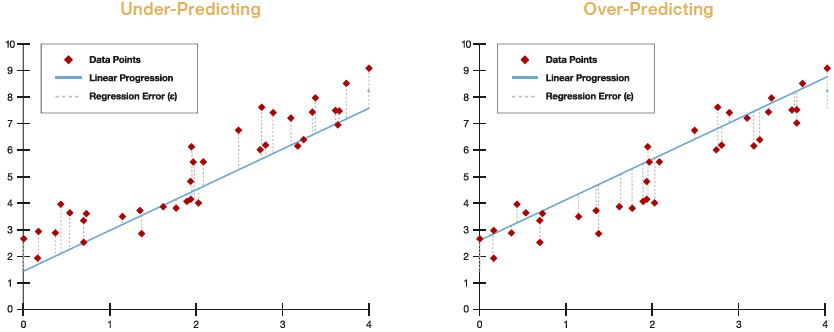# Tuning a Machine Learning Model

I continue taking some basic notes of the book “Enterprise Artificial Intelligence and Machine Learning for Managers“.

Tuning a machine learning model is an iterative process. Data scientists typically run
numerous experiments to train and evaluate models, trying out different features,
different loss functions, different AI/ML models, and adjusting model parameters
and hyper-parameters.

# Feature engineering

Feature engineering broadly refers to mathematical transformations of raw data in order to feed appropriate signals into AI/ML models.

In real world data are derived from a variety of source systems and typically are not reconciled or aligned in time and space. Data scientists often put significant effort into defining data transformation pipelines and building out their feature vectors.

In addition, data scientists should implement requirements for feature normalization or scaling to ensure that no one feature overpowers the algorithm.

# Loss Functions

A loss function serves as the objective function that the AI/ML algorithm is seeking to optimize during training efforts. During model training, the AI/ML algorithm aims to minimize the loss function. Data scientists often consider different loss functions to
improve the model – e.g., make the model less sensitive to outliers, better handle noise, or reduce over-fitting.

A simple example of a loss function is mean squared error (MSE), which often is used to optimize regression models. MSE measures the average of squared difference between predictions and actual output values.These two linear regression models have the same MSE, but the model onthe left is under-predicting and the model on the right is over-predicting.

It is important, to recognize the weaknesses of loss functions. Over-relying on loss functions as an indicator of prediction accuracy may lead to erroneous model set points.

# Regularization

Regularization is a method to balance over-fitting and under-fitting a model during training. Both over-fitting and under-fitting are problems that ultimately cause poor predictions on new data.

• Over-fitting occurs when a machine learning model is tuned to learn the noise in the data rather than the patterns or trends in the data. A supervised model that is over-fit will typically perform well on data the model was trained on, but perform poorly on data the model has not seen before.
• Under-fitting occurs when the machine learning model does not capture variations in the data – where the variations in data are not caused by noise. Such a model is considered to have high bias, or low variance. A supervised model that is under-fit will typically perform poorly on both data the model was trained on, and
on data the model has not seen before.

Regularization is a technique to adjust how closely a model is trained to fit historical data. One way to apply regularization is by adding a parameter that penalizes the loss function when the tuned model is overfit.

# Hyper-parameters

Hyper-parameters are model parameters that are specified before training a model – i.e., parameters that are different from model parameters – or weights that an AI/ML model learns during model training.

Finding the best hyper-parameters is an iterative and potentially time intensive
process called “hyper-parameter optimization.”

Examples:

• Number of hidden layers and the learning rate of deep neural network algorithms.
• Number of leaves and depth of trees in decision tree algorithms.
• Number of clusters in clustering algorithms.

To address the challenge of hyper-parameter optimization, data scientists use specific optimization algorithms designed for this task (i.e.: grid search, random search, and Bayesian optimization). These optimization approaches help narrow the search
space of all possible hyper-parameter combinations to find the best (or near best) result.